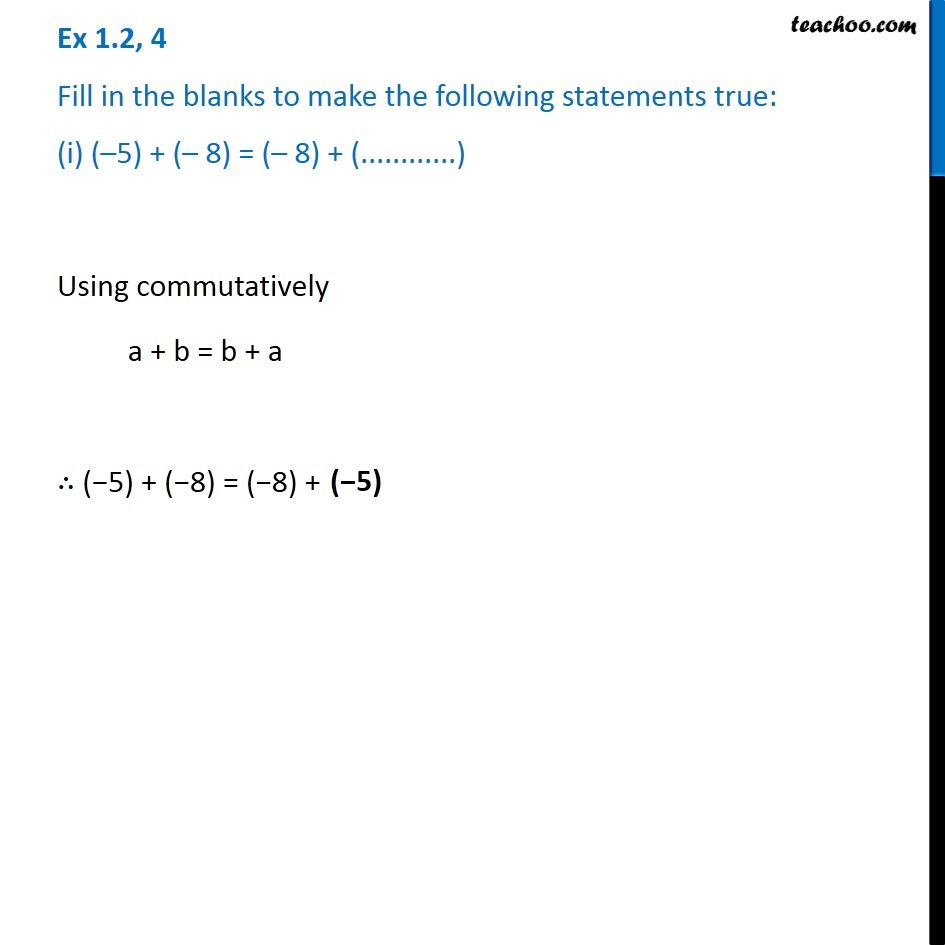1. Chapter 1 Class 7 Integers
2. Serial order wise
3. Ex 1.2

Transcript

Ex 1.2, 4 Fill in the blanks to make the following statements true: (i) (–5) + (– 8) = (– 8) + (............) Using commutatively a + b = b + a ∴ (−5) + (−8) = (−8) +(−5)

Ex 1.2

Chapter 1 Class 7 Integers
Serial order wise